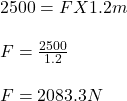## How much force is used if ms Johnston can bench press 123 kg in 3 seconds, using 2500 j of work? The total distance from the barbell to ms J

Question

How much force is used if ms Johnston can bench press 123 kg in 3 seconds, using 2500 j of work? The total distance from the barbell to ms Johnston to the original starting position is 1.2m.

in progress 0
6 months 2021-08-15T15:53:45+00:00 1 Answers 7 views 0

## Answers ( )

1. The force applied would be 2083.3N

Explanation:

Given:

Mass, m = 123kg

Time, t = 3 seconds

Work, W = 2500J

Distance, x = 1.2m

Force, F = ?

We know:

Work = Force X distanceTherefore, the force applied would be 2083.3N